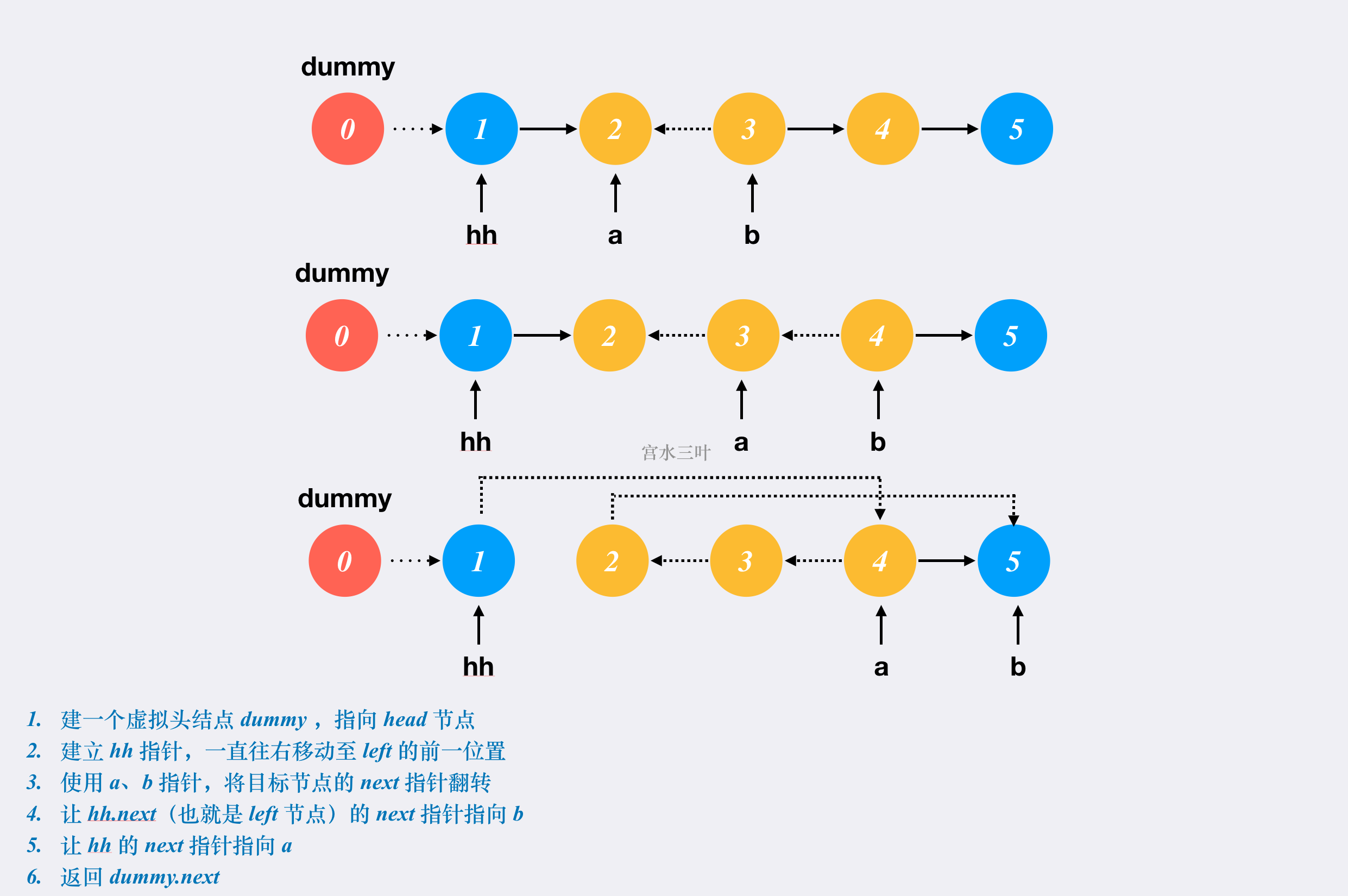# LC 92. 反转链表 II

### 题目描述• 链表中节点数目为 n
• 1 <= n <= 500
• -500 <= Node.val <= 500
• 1 <= left <= right <= n

### 朴素解法• 时间复杂度：$O(n)$
• 空间复杂度：$O(1)$